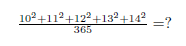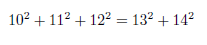Digital Tbucket Tank (DTT)

# Secrets of Algebra

The last article had a nice response (thanks for that). So today something from the world of "forgotten math" - have fun!

Arithmetic can often not prove some of its strongholds by vague means. In these cases we need more general algebra methods. For these types of arithmetic theorems, which are algebraically justified, there are many rules for abbreviated arithmetic operations.

Speed ​​multiplication:

In the old days without computers or calculators, great arithmeticists used many simple algebraic tricks; to make your life easier:

The "x" is representative of multiplication (we were too lazy to try LaTeX :-))

Let's look at:

988² =?

It's very simple, let's take a closer look:

988 x 988 = (988 + 12) x (998 -12) + 12² = 1000 x 976 + 144 = 976 144

It's also easy to understand what's going on here:

(a + b) (a - b) + b² = a² - b² + b² = a²

OK so far so good. Now let's try to do the math quickly - even combinations like

986 x 997, without calculator!

986 x 997 = (986 - 3) x 1000 + 3 x 14 = 983 042

What happened here? We can write down the factors as follows:

# A "difficult" problem

Today something from the category "forgotten math". There are always very interesting algebraic number relationships that are unfortunately rarely or not at all in the curriculum, but which expand the understanding of numbers and mathematical intuition.

Let's say someone asks you to solve the next equation without any technical tools.Can you do this?

Ok at first sight is not that easy. But when you know the special and interesting relationship between these numbers, it's really simple:The left components of the equation are: 100 + 121 + 144 = 365; In other words:Ok, let's use simple algebra to find out if we can find more such sequences: The first number we are looking for is "x":